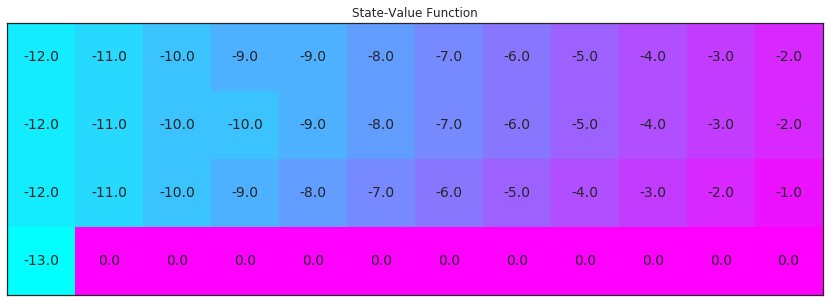# Mini Project: Temporal-Difference Methods¶

In this notebook, you will write your own implementations of many Temporal-Difference (TD) methods.

While we have provided some starter code, you are welcome to erase these hints and write your code from scratch.

### Part 0: Explore CliffWalkingEnv¶

Use the code cell below to create an instance of the CliffWalking environment.

In :
import gym
env = gym.make('CliffWalking-v0')


The agent moves through a $4\times 12$ gridworld, with states numbered as follows:

[[ 0,  1,  2,  3,  4,  5,  6,  7,  8,  9, 10, 11],
[12, 13, 14, 15, 16, 17, 18, 19, 20, 21, 22, 23],
[24, 25, 26, 27, 28, 29, 30, 31, 32, 33, 34, 35],
[36, 37, 38, 39, 40, 41, 42, 43, 44, 45, 46, 47]]

At the start of any episode, state 36 is the initial state. State 47 is the only terminal state, and the cliff corresponds to states 37 through 46.

The agent has 4 potential actions:

UP = 0
RIGHT = 1
DOWN = 2
LEFT = 3

Thus, $\mathcal{S}^+=\{0, 1, \ldots, 47\}$, and $\mathcal{A} =\{0, 1, 2, 3\}$. Verify this by running the code cell below.

In :
print(env.action_space)
print(env.observation_space)

Discrete(4)
Discrete(48)


In this mini-project, we will build towards finding the optimal policy for the CliffWalking environment. The optimal state-value function is visualized below. Please take the time now to make sure that you understand why this is the optimal state-value function.

In :
import numpy as np
from plot_utils import plot_values

# define the optimal state-value function
V_opt = np.zeros((4,12))
V_opt[0:13] = -np.arange(3, 15)[::-1]
V_opt[0:13] = -np.arange(3, 15)[::-1] + 1
V_opt[0:13] = -np.arange(3, 15)[::-1] + 2
V_opt = -13

plot_values(V_opt)

<matplotlib.figure.Figure at 0x7fa5a2e66c88>

### Part 1: TD Prediction: State Values¶

In this section, you will write your own implementation of TD prediction (for estimating the state-value function).

We will begin by investigating a policy where the agent moves:

• RIGHT in states 0 through 10, inclusive,
• DOWN in states 11, 23, and 35, and
• UP in states 12 through 22, inclusive, states 24 through 34, inclusive, and state 36.

The policy is specified and printed below. Note that states where the agent does not choose an action have been marked with -1.

In :
policy = np.hstack([1*np.ones(11), 2, 0, np.zeros(10), 2, 0, np.zeros(10), 2, 0, -1*np.ones(11)])
print("\nPolicy (UP = 0, RIGHT = 1, DOWN = 2, LEFT = 3, N/A = -1):")
print(policy.reshape(4,12))

Policy (UP = 0, RIGHT = 1, DOWN = 2, LEFT = 3, N/A = -1):
[[ 1.  1.  1.  1.  1.  1.  1.  1.  1.  1.  1.  2.]
[ 0.  0.  0.  0.  0.  0.  0.  0.  0.  0.  0.  2.]
[ 0.  0.  0.  0.  0.  0.  0.  0.  0.  0.  0.  2.]
[ 0. -1. -1. -1. -1. -1. -1. -1. -1. -1. -1. -1.]]


Run the next cell to visualize the state-value function that corresponds to this policy. Make sure that you take the time to understand why this is the corresponding value function!

In :
V_true = np.zeros((4,12))
for i in range(3):
V_true[0:12][i] = -np.arange(3, 15)[::-1] - i
V_true = -2
V_true = -1
V_true = -17

plot_values(V_true)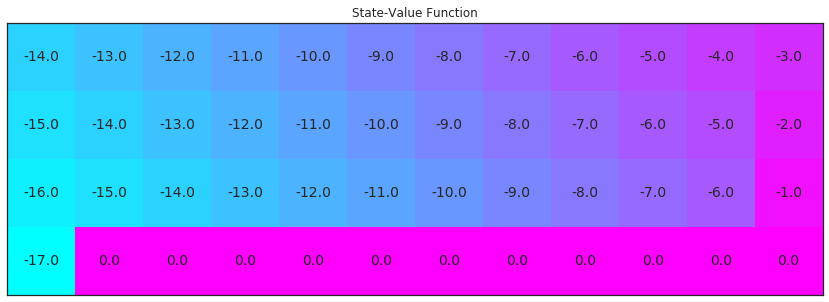The above figure is what you will try to approximate through the TD prediction algorithm.

Your algorithm for TD prediction has five arguments:

• env: This is an instance of an OpenAI Gym environment.
• num_episodes: This is the number of episodes that are generated through agent-environment interaction.
• policy: This is a 1D numpy array with policy.shape equal to the number of states (env.nS). policy[s] returns the action that the agent chooses when in state s.
• alpha: This is the step-size parameter for the update step.
• gamma: This is the discount rate. It must be a value between 0 and 1, inclusive (default value: 1).

The algorithm returns as output:

• V: This is a dictionary where V[s] is the estimated value of state s.

Please complete the function in the code cell below.

In :
# In this cell I am just getting comfortable with the environement

# trying the first step:
# - resets the state to the starting point
# - then get the action from the policy
# - give the action to the environments step function and see what you get

print("--> trying first steps:")

# reset the environment
state = env.reset()
print("state:", state)

# get the action from the policy
action = policy[state]
print("action:", action)

# perform a step on the environment with that action
step = env.step(action)
print("resulting step:", step)

# running a full episode
# do the above until the step brings back, that we are done
print("\n--> now running a full episode:")

# reset the environment
state = env.reset()
while True:
# perform the next step
action = policy[state]
state, reward, episode_end, probs = env.step(action)
print(state, reward, episode_end, probs)

# break when the episode ended
if episode_end:
break

--> trying first steps:
state: 36
action: 0.0
resulting step: (24, -1, False, {'prob': 1.0})

--> now running a full episode:
24 -1 False {'prob': 1.0}
12 -1 False {'prob': 1.0}
0 -1 False {'prob': 1.0}
1 -1 False {'prob': 1.0}
2 -1 False {'prob': 1.0}
3 -1 False {'prob': 1.0}
4 -1 False {'prob': 1.0}
5 -1 False {'prob': 1.0}
6 -1 False {'prob': 1.0}
7 -1 False {'prob': 1.0}
8 -1 False {'prob': 1.0}
9 -1 False {'prob': 1.0}
10 -1 False {'prob': 1.0}
11 -1 False {'prob': 1.0}
23 -1 False {'prob': 1.0}
35 -1 False {'prob': 1.0}
47 -1 True {'prob': 1.0}

In :
from collections import defaultdict, deque
import sys

def td_prediction(env, num_episodes, policy, alpha, gamma=1.0):
"""
this function approximates the state function by interacting with the environment

for a fixed number of episodes the state function is improved in each step
"""
# initialize V as a dictionaries of floats with values 0
V = defaultdict(float)

# loop over episodes
for i_episode in range(1, num_episodes+1):

# monitor progress
if i_episode % 100 == 0:
print("\rEpisode {}/{}".format(i_episode, num_episodes), end="")
sys.stdout.flush()

# start the episode by resetting the environment
state = env.reset()

# go through the episode
while True:
# perform the next step
action = policy[state]
next_state, reward, episode_end, _ = env.step(action)

# now immediately update the state function in every step with that
# steps reward + the discounted next steps reward
# use what ever state function you have for each state to calculate the
# updata
V[state] = (1 - alpha) * V[state] + alpha * (reward + gamma * V[next_state])

# set the state to the next state in order to continue the episode
state = next_state

# check whether the episode ended
if episode_end:
break

# finally return the approximated state function for the policy
return V


Run the code cell below to test your implementation and visualize the estimated state-value function. If the code cell returns PASSED, then you have implemented the function correctly! Feel free to change the num_episodes and alpha parameters that are supplied to the function. However, if you'd like to ensure the accuracy of the unit test, please do not change the value of gamma from the default.

In :
import check_test

# evaluate the policy and reshape the state-value function
V_pred = td_prediction(env, 5000, policy, .01)

# please do not change the code below this line
V_pred_plot = np.reshape([V_pred[key] if key in V_pred else 0 for key in np.arange(48)], (4,12))
check_test.run_check('td_prediction_check', V_pred_plot)
plot_values(V_pred_plot)

Episode 5000/5000

PASSED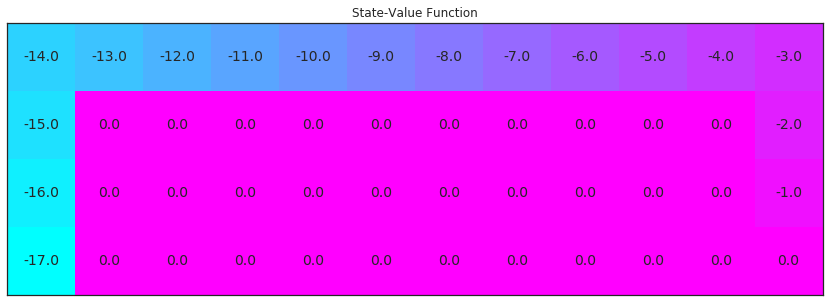How close is your estimated state-value function to the true state-value function corresponding to the policy?

You might notice that some of the state values are not estimated by the agent. This is because under this policy, the agent will not visit all of the states. In the TD prediction algorithm, the agent can only estimate the values corresponding to states that are visited.

### Part 2: TD Control: Sarsa¶

In this section, you will write your own implementation of the Sarsa control algorithm.

Your algorithm has four arguments:

• env: This is an instance of an OpenAI Gym environment.
• num_episodes: This is the number of episodes that are generated through agent-environment interaction.
• alpha: This is the step-size parameter for the update step.
• gamma: This is the discount rate. It must be a value between 0 and 1, inclusive (default value: 1).

The algorithm returns as output:

• Q: This is a dictionary (of one-dimensional arrays) where Q[s][a] is the estimated action value corresponding to state s and action a.

Please complete the function in the code cell below.

[[ 0,  1,  2,  3,  4,  5,  6,  7,  8,  9, 10, 11],
[12, 13, 14, 15, 16, 17, 18, 19, 20, 21, 22, 23],
[24, 25, 26, 27, 28, 29, 30, 31, 32, 33, 34, 35],
[36, 37, 38, 39, 40, 41, 42, 43, 44, 45, 46, 47]]
In :
def Q_update(Q_S1_A1, Q_S2_A2, reward, alpha, gamma):
""" updates the action-value function estimate using the most recent time step """
return Q_S1_A1 * (1 - alpha) + (reward + gamma * Q_S2_A2) * alpha

def get_epsilon_greedy_probs(env, Q_S, epsilon):
""" obtains the action probabilities corresponding to epsilon-greedy policy """
probs = np.ones(env.nA) * epsilon / env.nA

mask = Q_S == np.max(Q_S)
greedy_count = len(greedy_choices)

probs[greedy_choices] = \
(1 - ((env.nA - greedy_count) * epsilon / env.nA)) / greedy_count
return probs

In :
def sarsa(env, num_episodes, alpha, gamma=1.0):

# initialize action-value as default dict of maps
Q = defaultdict(lambda: np.zeros(env.nA))

# loop over episodes
for i_episode in range(1, num_episodes + 1):

# monitor progress
if i_episode % 100 == 0:
print("\rEpisode {}/{}".format(i_episode, num_episodes), end="")
sys.stdout.flush()

# set epsilon
epsilon = 1 / ((i_episode + 1) * 10)

# start episode
S1 = env.reset()

# pick action A1
A1 = np.random.choice(
np.arange(env.nA),
p=get_epsilon_greedy_probs(env, Q[S1], epsilon))
# limit number of time steps per episode

for t_step in np.arange(300):
# take action A1, observe R, S2
S2, reward, done, info = env.step(A1)

if not done:
# pick next action A2
A2 = np.random.choice(
np.arange(env.nA),
p=get_epsilon_greedy_probs(env, Q[S2], epsilon))

# update Q
Q[S1][A1] = Q_update(Q[S1][A1], Q[S2][A2],
reward, alpha, gamma)
# S1, A1 = S2, A2
S1, A1 = S2, A2

if done:
# update TD estimate of Q
Q[S1][A1] = Q_update(Q[S1][A1], 0, reward, alpha, gamma)

break

return Q


Use the next code cell to visualize the estimated optimal policy and the corresponding state-value function.

If the code cell returns PASSED, then you have implemented the function correctly! Feel free to change the num_episodes and alpha parameters that are supplied to the function. However, if you'd like to ensure the accuracy of the unit test, please do not change the value of gamma from the default.

In :
# obtain the estimated optimal policy and corresponding action-value function
Q_sarsa = sarsa(env, 5000, .01)

# print the estimated optimal policy
policy_sarsa = np.array([np.argmax(Q_sarsa[key]) if key in Q_sarsa else -1 for key in np.arange(48)]).reshape(4,12)
check_test.run_check('td_control_check', policy_sarsa)
print("\nEstimated Optimal Policy (UP = 0, RIGHT = 1, DOWN = 2, LEFT = 3, N/A = -1):")
print(policy_sarsa)

# plot the estimated optimal state-value function
V_sarsa = ([np.max(Q_sarsa[key]) if key in Q_sarsa else 0 for key in np.arange(48)])
plot_values(V_sarsa)

Episode 5000/5000

PASSED

Estimated Optimal Policy (UP = 0, RIGHT = 1, DOWN = 2, LEFT = 3, N/A = -1):
[[ 1  1  3  1  2  1  0  1  1  1  1  2]
[ 1  3  1  1  1  1  2  0  1  1  1  2]
[ 1  1  1  1  1  1  1  1  1  1  1  2]
[ 0 -1 -1 -1 -1 -1 -1 -1 -1 -1 -1 -1]]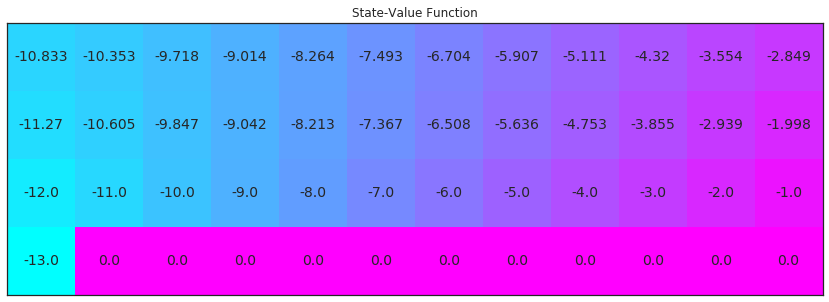### Part 3: TD Control: Q-learning¶

In this section, you will write your own implementation of the Q-learning control algorithm.

Your algorithm has four arguments:

• env: This is an instance of an OpenAI Gym environment.
• num_episodes: This is the number of episodes that are generated through agent-environment interaction.
• alpha: This is the step-size parameter for the update step.
• gamma: This is the discount rate. It must be a value between 0 and 1, inclusive (default value: 1).

The algorithm returns as output:

• Q: This is a dictionary (of one-dimensional arrays) where Q[s][a] is the estimated action value corresponding to state s and action a.

Please complete the function in the code cell below.

In :
def get_greedy_probs(env, Q_S):
""" obtains the action probabilities corresponding to epsilon-greedy policy """
probs = np.zeros(env.nA)

mask = Q_S == np.max(Q_S)
greedy_count = len(greedy_choices)

probs[greedy_choices] = 1 / greedy_count

return probs

In :
def q_learning(env, num_episodes, alpha, gamma=1.0):
# initialize empty dictionary of arrays
Q = defaultdict(lambda: np.zeros(env.nA))
# loop over episodes
for i_episode in range(1, num_episodes+1):
# monitor progress
if i_episode % 100 == 0:
print("\rEpisode {}/{}".format(i_episode, num_episodes), end="")
sys.stdout.flush()

# set epsilon
epsilon = 1 / ((i_episode + 1) * 10)

# start episode
S1 = env.reset()

# pick action A1
A1 = np.random.choice(
np.arange(env.nA),
p=get_epsilon_greedy_probs(env, Q[S1], epsilon))
# limit number of time steps per episode

for t_step in np.arange(300):
# take action A1, observe R, S2
S2, reward, done, info = env.step(A1)

if not done:

# pick greedy action to update
A2_greedy = np.random.choice(
np.arange(env.nA),
p=get_greedy_probs(env, Q[S2]))

# update Q
Q[S1][A1] = Q_update(Q[S1][A1], Q[S2][A2_greedy],
reward, alpha, gamma)

# pick next action A2
A2 = np.random.choice(
np.arange(env.nA),
p=get_epsilon_greedy_probs(env, Q[S2], epsilon))

# S1, A1 = S2, A2
S1, A1 = S2, A2

if done:
# update TD estimate of Q
Q[S1][A1] = Q_update(Q[S1][A1], 0, reward, alpha, gamma)

break

return Q


Use the next code cell to visualize the estimated optimal policy and the corresponding state-value function.

If the code cell returns PASSED, then you have implemented the function correctly! Feel free to change the num_episodes and alpha parameters that are supplied to the function. However, if you'd like to ensure the accuracy of the unit test, please do not change the value of gamma from the default.

In :
# obtain the estimated optimal policy and corresponding action-value function
Q_sarsamax = q_learning(env, 5000, .01)

# print the estimated optimal policy
policy_sarsamax = np.array([np.argmax(Q_sarsamax[key]) if key in Q_sarsamax else -1 for key in np.arange(48)]).reshape((4,12))
check_test.run_check('td_control_check', policy_sarsamax)
print("\nEstimated Optimal Policy (UP = 0, RIGHT = 1, DOWN = 2, LEFT = 3, N/A = -1):")
print(policy_sarsamax)

# plot the estimated optimal state-value function
plot_values([np.max(Q_sarsamax[key]) if key in Q_sarsamax else 0 for key in np.arange(48)])

Episode 5000/5000

PASSED

Estimated Optimal Policy (UP = 0, RIGHT = 1, DOWN = 2, LEFT = 3, N/A = -1):
[[ 2  1  0  2  2  0  1  1  0  1  3  2]
[ 3  1  1  1  2  2  1  2  3  1  1  2]
[ 1  1  1  1  1  1  1  1  1  1  1  2]
[ 0 -1 -1 -1 -1 -1 -1 -1 -1 -1 -1 -1]]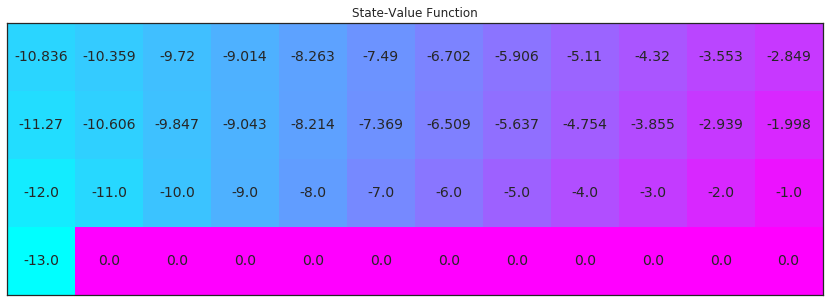### Part 4: TD Control: Expected Sarsa¶

In this section, you will write your own implementation of the Expected Sarsa control algorithm.

Your algorithm has four arguments:

• env: This is an instance of an OpenAI Gym environment.
• num_episodes: This is the number of episodes that are generated through agent-environment interaction.
• alpha: This is the step-size parameter for the update step.
• gamma: This is the discount rate. It must be a value between 0 and 1, inclusive (default value: 1).

The algorithm returns as output:

• Q: This is a dictionary (of one-dimensional arrays) where Q[s][a] is the estimated action value corresponding to state s and action a.

Please complete the function in the code cell below.

In :
def expected_sarsa(env, num_episodes, alpha, gamma=1.0):
# initialize empty dictionary of arrays
Q = defaultdict(lambda: np.zeros(env.nA))
# loop over episodes
for i_episode in range(1, num_episodes+1):
# monitor progress
if i_episode % 100 == 0:
print("\rEpisode {}/{}".format(i_episode, num_episodes), end="")
sys.stdout.flush()

# set epsilon
epsilon = 1 / ((i_episode + 1) * 10)

# start episode
S1 = env.reset()

# pick action A1
A1 = np.random.choice(
np.arange(env.nA),
p=get_epsilon_greedy_probs(env, Q[S1], epsilon))
# limit number of time steps per episode

for t_step in np.arange(300):
# take action A1, observe R, S2
S2, reward, done, info = env.step(A1)

if not done:

# pick greedy action to update
greedy_probs = get_greedy_probs(env, Q[S2])

# update Q
Q[S1][A1] = Q_update(Q[S1][A1], np.dot(Q[S2], greedy_probs),
reward, alpha, gamma)

# pick next action A2
A2 = np.random.choice(
np.arange(env.nA),
p=get_epsilon_greedy_probs(env, Q[S2], epsilon))

# S1, A1 = S2, A2
S1, A1 = S2, A2

if done:
# update TD estimate of Q
Q[S1][A1] = Q_update(Q[S1][A1], 0, reward, alpha, gamma)

break

return Q


Use the next code cell to visualize the estimated optimal policy and the corresponding state-value function.

If the code cell returns PASSED, then you have implemented the function correctly! Feel free to change the num_episodes and alpha parameters that are supplied to the function. However, if you'd like to ensure the accuracy of the unit test, please do not change the value of gamma from the default.

In :
# obtain the estimated optimal policy and corresponding action-value function
Q_expsarsa = expected_sarsa(env, 10000, 1)

# print the estimated optimal policy
policy_expsarsa = np.array([np.argmax(Q_expsarsa[key]) if key in Q_expsarsa else -1 for key in np.arange(48)]).reshape(4,12)
check_test.run_check('td_control_check', policy_expsarsa)
print("\nEstimated Optimal Policy (UP = 0, RIGHT = 1, DOWN = 2, LEFT = 3, N/A = -1):")
print(policy_expsarsa)

# plot the estimated optimal state-value function
plot_values([np.max(Q_expsarsa[key]) if key in Q_expsarsa else 0 for key in np.arange(48)])

Episode 10000/10000

PASSED

Estimated Optimal Policy (UP = 0, RIGHT = 1, DOWN = 2, LEFT = 3, N/A = -1):
[[ 0  0  1  1  1  1  0  1  1  0  1  2]
[ 0  1  1  0  1  1  0  0  0  0  1  2]
[ 1  1  1  1  1  1  1  1  1  1  1  2]
[ 0 -1 -1 -1 -1 -1 -1 -1 -1 -1 -1 -1]]